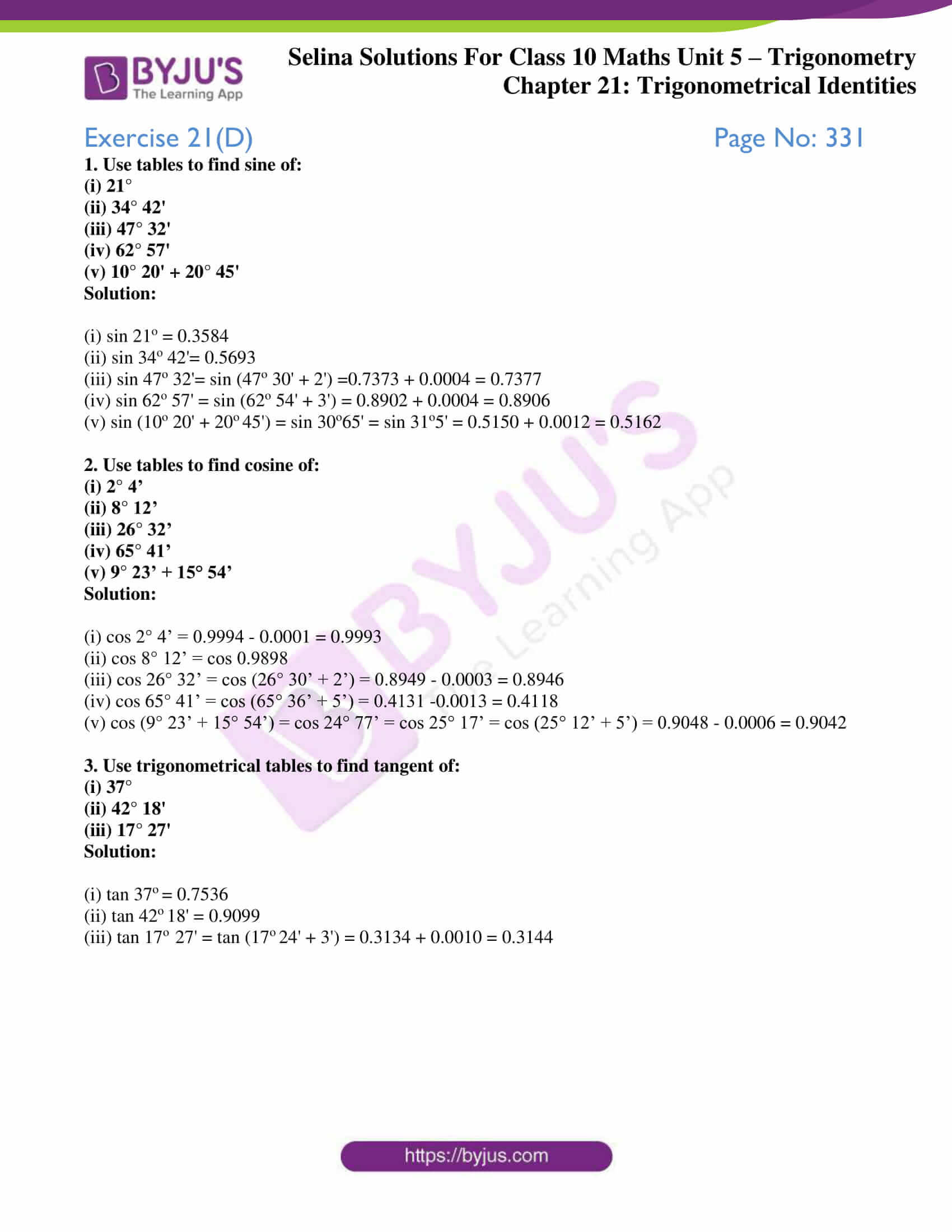# Selina Solutions Concise Maths Class 10 Chapter 21 Trigonometrical Identities Exercise 21(D)

The use of trigonometrical tables to find various trigonometric ratios of angles are practised in this exercise. Students wanting to know the right steps to solve problems can take a quick reference at the Selina Solutions for Class 10 Maths. The solutions are prepared by subject matter experts at BYJU’S, keeping in mind the latest ICSE marking schemes. The solutions PDF of the Concise Selina Solutions for Class 10 Maths Chapter 21 Trigonometrical Identities Exercise 21(D) can be accessed from the link below.

## Selina Solutions Concise Maths Class 10 Chapter 21 Trigonometrical Identities Exercise 21(D) Download PDF### Access Selina Solutions Concise Maths Class 10 Chapter 21 Trigonometrical Identities Exercise 21(D)

1. Use tables to find sine of:

(i) 21°

(ii) 34° 42′

(iii) 47° 32′

(iv) 62° 57′

(v) 10° 20′ + 20° 45′

Solution:

(i) sin 21o = 0.3584

(ii) sin 34o 42’= 0.5693

(iii) sin 47o 32’= sin (47o 30′ + 2′) =0.7373 + 0.0004 = 0.7377

(iv) sin 62o 57′ = sin (62o 54′ + 3′) = 0.8902 + 0.0004 = 0.8906

(v) sin (10o 20′ + 2045′) = sin 30o65′ = sin 31o5′ = 0.5150 + 0.0012 = 0.5162

2. Use tables to find cosine of:

(i) 2° 4’

(ii) 8° 12’

(iii) 26° 32’

(iv) 65° 41’

(v) 9° 23’ + 15° 54’

Solution:

(i) cos 2° 4’ = 0.9994 – 0.0001 = 0.9993

(ii) cos 8° 12’ = cos 0.9898

(iii) cos 26° 32’ = cos (26° 30’ + 2’) = 0.8949 – 0.0003 = 0.8946

(iv) cos 65° 41’ = cos (65° 36’ + 5’) = 0.4131 -0.0013 = 0.4118

(v) cos (9° 23’ + 15° 54’) = cos 24° 77’ = cos 25° 17’ = cos (25° 12’ + 5’) = 0.9048 – 0.0006 = 0.9042

3. Use trigonometrical tables to find tangent of:

(i) 37°

(ii) 42° 18′

(iii) 17° 27′

Solution:

(i) tan 37= 0.7536

(ii) tan 4218′ = 0.9099

(iii) tan 17o  27′ = tan (1724′ + 3′) = 0.3134 + 0.0010 = 0.3144